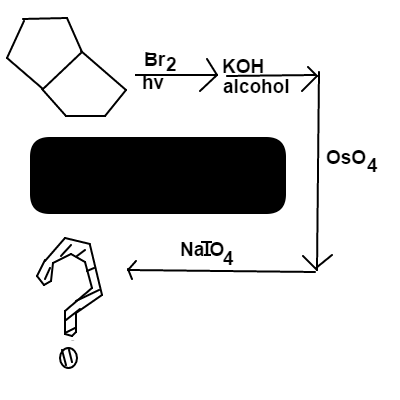# Bicyclo[3.3.0] Octane

Chemistry Level 3$\ce{Bicyclo[3}.\ce{3}.\ce{0] octane}$ is reacted in the following order:

• $\ce{Br}_2$ in $\ce{light}$

• $\ce{KOH}$ in $\ce{alcohol}$

• $\ce{OsO}_4$ ($\ce{Osmium Tetroxide}$)

• $\ce{NaIO}_4$ ($\ce{Sodium Periodate}$)

What will be the $\ce{degrees~of~unsaturation}$ for the $\ce{major~end~product}$?

David's Organic Chemistry Set

David's Physical Chemistry Set

×